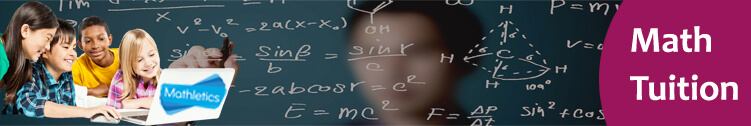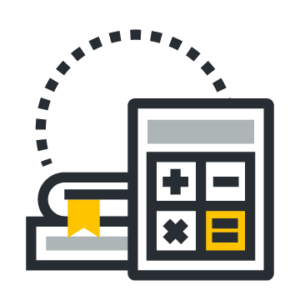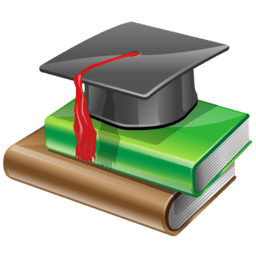## Secondary 4 Maths# Maths Tuition Center for Secondary Class 4

The purpose of this Maths Tuition Centre For Secondary 4 is to prepare students for the O level A math examination. The sole purpose of this course is to make students excel in mathematics. Students will be made to focus and do thorough revision of all key points by means of tests that will aim to check fundamental knowledge of students.

About the course- Students taking up this maths tuition course will be taught the following mentioned topics in the class.

• Students will be taught about various geometric theorems such as mid point theorem. Students will be taught how to write formal proofs and be able to justify the truthfulness of a geometric statement.
• Students will be introduced to the most difficult topic in secondary mathematics, the calculus.
• Students will be taught how to study real world problems formulate it in mathematical solutions in it real world context.
• Students will be taught how to recognize different types of questions and what kind of approach is required to solve what type of question.

Target students – This course is meant only for students of secondary class four both normal and express academic.

Why choose inspizone?

Students at inspizone are trained to grasp the concepts and retain them for a long time by teaching them in a very creative and interactive way as they get advantage of other benefits mentioned below as well.

• Students are taught in small class sizes that help them to easily communicate with teachers and get a better understanding of concepts
• Students are provided with quality study material that can be referred to at any time.
• Students are assessed and monitored constantly that help them to recognize their strong and weak points and work upon them thereby.

Schedule: Weekdays (Monday to Friday: 5.30 pm to 8.30 pm)
Saturday and Sunday: 9.30 am to 4 pm.

Venue: 10 Anson Road, 26-08A International Plaza, Singapore 079903

##Secondary 4 School Mathematics (Syllabus)

### Set Theory

• Set Language and Notation

• Probability

### Statistics

• Data Analysis

### Vectors

• Vectors in Two Dimensions

### Matrices

• Matrices and Their Applications

### Plane Geometry

• Triangle Theorem
• Quadrilateral Theorem
• Circle theorem

### Trigonometric Functions

• Trigonometric Ratios of Acute Angles
• Trigonometric Ratios of Any Angles
• Graphs of Trigonometric Functions

### Simple Trigonometric Identities and Equations

• Simple Identities
• Further Trigonometric Equations

### Further Trigonometric Identities

• The Addition Formulae
• The Double Angle Formulae
• The R-Formulae

### Differentiation

• The Derivative and its Basic Rules
• The Chain Rule
• The product Rule
• The Quotient Rule

### Tangent, Normal and Rate of Change

• Equations of Tangents and Normals
• Increasing and Decreasing Functions
• Rates of Change
• Connected Rates of Change

### Maxima and Minima

• Nature of Stationary Points
• Maxima and minima Problems

### Further Differentiation

• Derivatives of Trigonometric Functions
• Derivatives of Exponential Functions
• Derivatives of Logarithmic Functions

### Integration

• Indefinite Integrals
• Definite Integrals
• Integration of Trigonometric Functions
• Integration of Exponential Functions and

### Application of Integration – Area of a Region

• Area Between a Curve and an Axis
• Area between a Curve and a Line

### Application of Integration – Kinematics

• Displacement Velocity and Acceleration

##Why Choose Inspigenius?

• Small Class Size: Focus on each student’s Performance
• Motivate: Bring out the student’s creativity.
• Engage: Engage students in Study and better learning environment.
• Interactivity: Interactive Class with technology and latest pedagogy
• Review: Review the learning and chart student’s performance.
• Assessment: Free Monthly Assessment
• Teachers: Experienced teacher with the right ethos and methodology
• Study Materials: Free comprehensive courses material